### Create an Account

Already have account?

### Forgot Your Password ?

Home / Questions / Determine the current through R in the circuit of Figure P7 66 v1 170 cos ωt V v...

# Determine the current through R in the circuit of Figure P7 66 v1 170 cos ωt V v2 170 cosωt 2π3 V v3

Determine the current through in the circuit of

Figure P7.66:

v1  = 170 cost)      V

v2  = 170 cos− 2π/3)    v3  = 170 cos+ 2π/3)      f  = 400 Hz         = 100 Q

C  = 0.47 μF            = 100 mH

V1                                                                                                             V2                                                                                     V3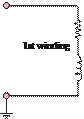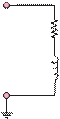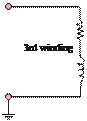R1                                           R2                                                       R3

2nd winding

L1                                           L2                                                       L3

(a)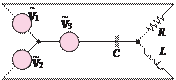~                                    ~                        ~V1                                                                                                       V2                                                                     V3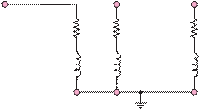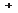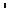R1               R2                                  R3

Jun 18 2020 View more View Less

#### Answer (Solved)Subscribe To Get Solution